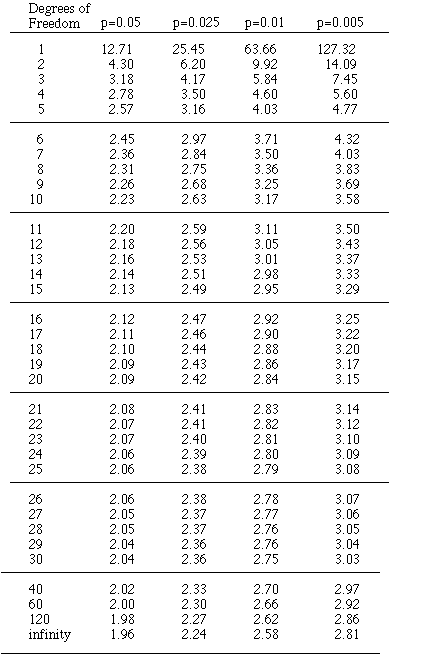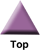### Home

Laboratory
Studies

Recordkeeping, Writing,
& Data Analysis

Laboratory
Methods

Overview
Microscope studies

Flagella experiment
Laboratory math
Blood fractionation
Gel electrophoresis
Protein gel analysis
Mitochondria
Concepts/ theory
Overview
Keeping a lab notebook
Writing research papers
Dimensions & units
Using figures (graphs)
Examples of graphs
Experimental error
Representing error
Applying statistics
Overview
Principles of microscopy

Solutions & dilutions
Protein assays
Spectrophotometry
Fractionation & centrifugation

# Selected Critical Values of the t-Distribution

A test is 2-tailed if you ask the question, 'does population 1 differ from population 2'. Then, if the mean for population 1 is significantly greater or smaller than that for population 2, you reject the null hypothesis. If you ask simply, is the true mean for population 1 greater than that for population 2, then you reject the null hypothesis only if the experimental mean for population 1 is found to be significantly greater. It can be significantly smaller, but you weren't asking that question. That would be a one-tailed test. For a two-tailed test if the calculated value of t exceeds the tabled value, then report the p value in the table. For a one-tailed test, the p value is divided by two. So 'p < 0.05' becomes 'p < 0.025."

The table should include values for p=0.1 so that a one-tailed test can be conducted at the p=0.05 level, but we never do such tests in my class, so why clutter up the table?